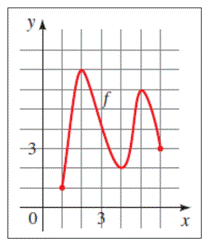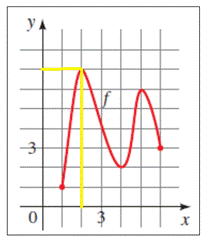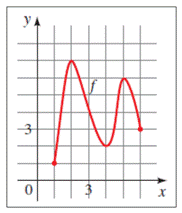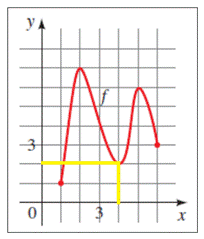# To fill in the gaps : with reference to the provided graph### Precalculus: Mathematics for Calcu...

6th Edition
Stewart + 5 others
Publisher: Cengage Learning
ISBN: 9780840068071### Precalculus: Mathematics for Calcu...

6th Edition
Stewart + 5 others
Publisher: Cengage Learning
ISBN: 9780840068071

#### Solutions

Chapter 2.3, Problem 4E

a.

To determine

## To fill in the gaps: with reference to the provided graph

Expert Solution

A function value f ( a ) is a local maximum value of f if f ( a ) is the highest value of f on some interval containing a . From the graph of f , we see that one local maximum value of f is 7 and that this value occurs when x is 2 .

### Explanation of Solution

Given information: A function value f ( a ) is a local maximum value of f if f ( a ) is the ______ value of f on some interval containing a . From the graph of f , we see that one local maximum value of f is _____ and that this value occurs when x is ______.Graph:

From the provided information, consider,Interpretation:

Local maximum value of f ( a ) on a graph of f is the highest value on an interval containing a . Therefore, the local maximum value on the provided graph of f is 7, at x = 2.

Hence, a function value f ( a ) is a local maximum value of f if f ( a ) is the highest value of f on some interval containing a . From the graph of f , we see that one local maximum value of f is 7 and that this value occurs when x is 2 .

b.

To determine

### To fill in the gaps: with reference to the provided graph

Expert Solution

A function value f ( a ) is a local minimum value of f if f ( a ) is the lowest value of f on some interval containing a . From the graph of f , we see that one local minimum value of f is 2 and that this value occurs when x is 4 .

### Explanation of Solution

Given information: A function value f ( a ) is a local minimum value of f if f ( a ) is the ______ value of f on some interval containing a . From the graph of f , we see that one local minimum value of f is _____ and that this value occurs when x is ______.Graph:

From the provided information, consider,Interpretation:

The local minimum value of f ( a ) on a graph of f is the lowest value on an interval containing a . The local minimum value on the provided graph is 2, at x = 4.

Hence, a function value f ( a ) is a local minimum value of f if f ( a ) is the lowest value of f on some interval containing a . From the graph of f , we see that one local minimum value of f is 2 and that this value occurs when x is 4 .

### Have a homework question?

Subscribe to bartleby learn! Ask subject matter experts 30 homework questions each month. Plus, you’ll have access to millions of step-by-step textbook answers!